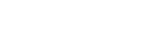## PHYSICS PAPER 1 Marking Scheme - 2017 KITUI MOCK EXAMINATION

SECTION A

1. M. S = 8.00
T.S = 0.30
= 8.30mm (Unit a must)
1. Tube A–because air molecules move faster than water molecules
2.
• Upthrust
• Frictional force
1. Let the point be x cm from pivot to the left side
Clockwise movement = anticlockwise moment
(x × 1) + 0.12(50 + x) = (50 – x) 0.18
x + 6 + 0.12x = 9 – 0.18x
1.3x = 3
x = 2.307cm
Answer = 50 – 2.307 = 47.69 cm (4 sf)
1.2. To prevent skidding or overturning of vehicles at high speed.
3. P = Fd/

=80×10×10×0.2

= 320W
1. When fire breaks mercury is heated. It expands and makes contact with terminal T, thereby completing the circuit and the bell rings.
2. k = F/e or series k = W/4÷2
=(W/8) mm
Total extension = F/k
e = (w/w/s)= 8mm
1.
• To remove air from the bottle
• Pressure imbalance on the inside and outside causes the higher atmospheric pressure on the outside
• The water drives air out of the bottle. On cooling, the steam inside condenses creating a region of low pressure inside. The higher atmospheric pressure deforms the bottle.
1. Rate of change of angular displacement with time.
2. Make building stable by lowering the centre of gravity
3. Pressure is inversely proportional to the speed or speed increases as pressure decreased

SECTION B

1.
1.
1. P.E = mgh
= 2 x 10 x 0.15
= 3J
2.2.
1. M1V1 + M2V2 = (M1 + M2)V
1 x 1 + 2 x 0 = (1 + 2)V
1 = 3V
V = 0.3333 ms-1
2.  K.E = ½ mv2
= ½ x 3 x 0.33332
= 0.495J
3.3. Velocity reduces to zero then increases to maximum value. The body is thrown upwards and coming back where it was released from.
1.
1. Bodies in circular motion change the direction at different instant implying a change of  velocity thus accelerates
2.
1. V = rw
w =v/r
v =2/2 = 1ms-1
2. w = 2πf/T, f=w/2π T=2π /w
=2π /0.2
= 31.42 seconds
3. T = mg + mrw2
= 0.04 x 10 + 0.04 x 0.5 x (0.2)2
= 0.4 + 0.0008N
= 0.4008N
4. As the clothes rotates in the spin driver, the fibre of the clothes cannotü1 provide sufficient centripetal force for the most of the water to make it go round in a circle. The water tends to fly off at a tangent to the circle and so move towards the wall where it gets out through the holes
5.
• Mass
• Velocity
1.
1. C is the quantity of heat required to change any mass of substance by 1oC or 1k. Whole s.h.c in the heat required to change a unit mass of substance by 1k.
2.
1. Time, temperature rise, current (I) and p.d (V), mass of liquid, mass of calorimeter and its contents               (Any 4 correct (2 marks
2. After t seconds, the new temperature is noted from the initial temperature of liquid.
Corresponding I and p.d should beü1 With the other variables the specific heat capacity in obtained from3. Ensure continuous stirring of the liquid.
3. pt = 0.4 x 900 x 10 + 1 x4200 x 10
2000t = 3600 + 42000
t =45600/2000
= 22.8 seconds
2. Product of force and distance moved by an object.
SI unit – Joule or NM
1. W   = mgh
= 500 x 10 x 4
= 20000J
2. P = w/t=20000/8
= 2,500w
3. power output/power inputx100%
=2500/2800×100
= 89.29%
4. Friction of the moving parts
Work done in lifting the parts of the machine
1.
1.
1. L = 3.0cm
=4/0.6
=6.667 cm/N
3. 1/slope
= 1/6.667
=0.15 N/cm or 15 N/m
2. w = wair - u
= 25 – 14
= 13N
3. Weight of stone aloneü1 is greater than upthrust, however stone and wood have smaller weight than upthrust

#### Download PHYSICS PAPER 1 Marking Scheme - 2017 KITUI MOCK EXAMINATION.

• ✔ To read offline at any time.
• ✔ To Print at your convenience
• ✔ Share Easily with Friends / Students